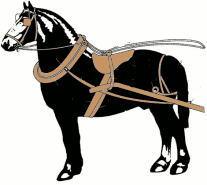WORKSHEET: ALGEBRA 3 - SOLUTION EXERCISE 9 9) A man buys a saddle, a horse, and a harness, for 360. The saddle costs twice as much as the harness ; and the horse costs twice as much as the harness and saddle together. What is the price of each?x - price of the harness 2x - price of the saddle 2(x+2x) - price of the horse x + 2x + 2(x + 2x)= 360 x + 2x + 6x = 360 9x = 360 x = 360:9 x = 40 Price of the harness - 40 Price of the saddle - 80 Price of the horse - 240 Return